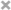# 那位先生

### 1.索引基础

#### 4.高性能的索引策略

``````select count(distinct left(name,3))/count(*) as sel3,
count(distinct left(name,4))/count(*) as sel4,
count(distinct left(name,5))/count(*) as sel5
from student;
``````

``````CREATE TABLE tb_test_index(
`id` INT NOT NULL PRIMARY KEY AUTO_INCREMENT,
`age` INT(11) DEFAULT NULL,
`birth` timestamp not NULL
)ENGINE=INNODB AUTO_INCREMENT=1 DEFAULT CHARSET=utf8;
alter table tb_test_index add index idx_age_bir(age,birth);
``````
``````explain select *from tb_test_index where 1=1 order by age,birth;	Using index;

explain select *from tb_test_index where 1=1 order by age;	Using index;

explain select *from tb_test_index where 1=1 order by birth;	Using index; Using filesort;

explain select *from tb_test_index where age = 10 order by birth;	Using where; Using index;

explain select *from tb_test_index where age > 10 order by birth;	Using where; Using index; Using filesort

explain select *from tb_test_index where age >10 order by age;	Using where; Using index

explain select *from tb_test_index where birth >10 order by age;	Using where; Using index

explain select *from tb_test_index where age >10 order by age,birth;	Using where; Using index

explain select *from tb_test_index where age >10 order by age asc,birth;	Using where; Using index

explain select *from tb_test_index where age >10 order by age asc,birth desc;	Using where; Using index; Using filesort

explain select *from tb_test_index where age >10 order by age desc,birth;	Using where; Using index; Using filesort

explain select *from tb_test_index where age >10 order by age desc,birth desc;	Using where; Using index
``````

#### 5.日期和时间类型

MySQL提供了两种相似的日期类型：DATETIME和TIMESTAMP。MySQL能存储的最小粒度为秒。
DATETIME：能保存最大范围的值为：1001年~9999年，精度为秒。它把日期和时间封装到格式为YYYYMMDDHHMMSS的整数中，与时区无关，使用8个字节的存储空间。 TIMESTAMP：保存了从1970年1月1日午夜（格林尼治时间）以来的秒数，它和UNIX时间戳相同，使用4个字节的存储空间。因为它的范围比DATETIME小：1970年~2038年。
TIMESTAMP提供的值和时区有关，而DATETIME则保留文本表示的日期和时间。除特殊情况外，通常尽量使用TIMESTAMP，因为相比DATETIME更节省空间。

### 3.范式和反范式化

#### 3.混用范式化和反范式化